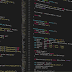//Program to search a number in any integer array using user defined function
#include<iostream.h>
#include<conio.h>
void SEARCH (int a,int n,int elements);
void main()
{clrscr();
int a,n, elements,i;
cout<<"\n Enter No. Of elements :";
cin>>n;
for (i=0;i<n;i++)
{cout<<"\n Enter a["<<i<<"] elements :";
cin>>a[i];
}
cout<<"\n The Array is :\n ";
for (i=0;i<n;i++)
cout<<a[i]<<" ";
cout<<"\n Enter The element to be search :";
cin>>elements;
SEARCH (a,n, elements);
getch();
}
void SEARCH (int a, int n ,int elements)
{int i, flag= 0;
for(i=0; i<n; i++)
{if ( a[i]== elements)
{flag=1;
break;
}
}
if(flag==0)
cout<<"\n Unsuccessful Search ";
else
cout<<"\n element is found at index "<<i<<" and at position "<<(i+1);
}
Program to search a number in any integer array using user defined functionReviewed by SHERE KHOJRAHA on February 16, 2019 Rating: 5